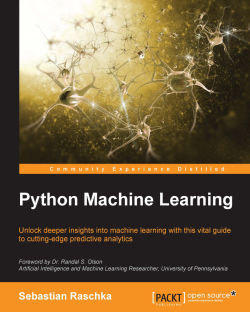# Python Machine Learning4.6 (107 reviews total)
By Sebastian Raschka
What do you get with a Packt Subscription?

• Constantly updated with 100+ new titles each month
• Breadth and depth in over 1,000+ technologies

Machine learning is transforming the way businesses operate. Being able to understand trends and patterns in complex data is critical to success; it is today one of the key strategies for unlocking growth in a challenging contemporary marketplace. Python can help you deliver key insights into your data – its unique capabilities as a language let you build sophisticated algorithms and statistical models that can reveal new perspectives and answer key questions that are vital for success.

Python Machine Learning gives you access to the world of machine learning and demonstrates why Python is one of the world’s leading data science languages. If you want to ask better questions of data, or need to improve and extend the capabilities of your machine learning systems, this practical data science book is invaluable. Covering a wide range of powerful Python libraries, including scikit-learn, Theano, and Keras, and featuring guidance and tips on everything from sentiment analysis to neural networks, you’ll soon be able to answer some of the most important questions facing you and your organization.

Publication date:
September 2015
Publisher
Packt
Pages
454
ISBN
9781783555130

## Chapter 1. Giving Computers the Ability to Learn from Data

In my opinion, machine learning, the application and science of algorithms that makes sense of data, is the most exciting field of all the computer sciences! We are living in an age where data comes in abundance; using the self-learning algorithms from the field of machine learning, we can turn this data into knowledge. Thanks to the many powerful open source libraries that have been developed in recent years, there has probably never been a better time to break into the machine learning field and learn how to utilize powerful algorithms to spot patterns in data and make predictions about future events.

In this chapter, we will learn about the main concepts and different types of machine learning. Together with a basic introduction to the relevant terminology, we will lay the groundwork for successfully using machine learning techniques for practical problem solving.

In this chapter, we will cover the following topics:

• The general concepts of machine learning

• The three types of learning and basic terminology

• The building blocks for successfully designing machine learning systems

• Installing and setting up Python for data analysis and machine learning

## Building intelligent machines to transform data into knowledge

In this age of modern technology, there is one resource that we have in abundance: a large amount of structured and unstructured data. In the second half of the twentieth century, machine learning evolved as a subfield of artificial intelligence that involved the development of self-learning algorithms to gain knowledge from that data in order to make predictions. Instead of requiring humans to manually derive rules and build models from analyzing large amounts of data, machine learning offers a more efficient alternative for capturing the knowledge in data to gradually improve the performance of predictive models, and make data-driven decisions. Not only is machine learning becoming increasingly important in computer science research but it also plays an ever greater role in our everyday life. Thanks to machine learning, we enjoy robust e-mail spam filters, convenient text and voice recognition software, reliable Web search engines, challenging chess players, and, hopefully soon, safe and efficient self-driving cars.

## The three different types of machine learning

In this section, we will take a look at the three types of machine learning: supervised learning, unsupervised learning, and reinforcement learning. We will learn about the fundamental differences between the three different learning types and, using conceptual examples, we will develop an intuition for the practical problem domains where these can be applied: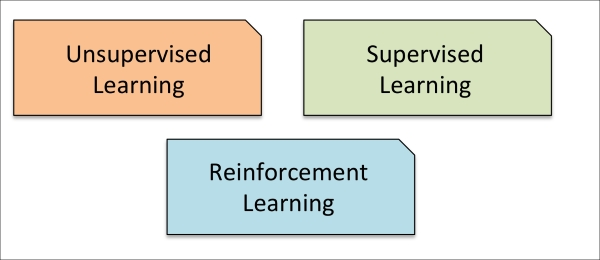### Making predictions about the future with supervised learning

The main goal in supervised learning is to learn a model from labeled training data that allows us to make predictions about unseen or future data. Here, the term supervised refers to a set of samples where the desired output signals (labels) are already known.

Considering the example of e-mail spam filtering, we can train a model using a supervised machine learning algorithm on a corpus of labeled e-mail, e-mail that are correctly marked as spam or not-spam, to predict whether a new e-mail belongs to either of the two categories. A supervised learning task with discrete class labels, such as in the previous e-mail spam-filtering example, is also called a classification task. Another subcategory of supervised learning is regression, where the outcome signal is a continuous value: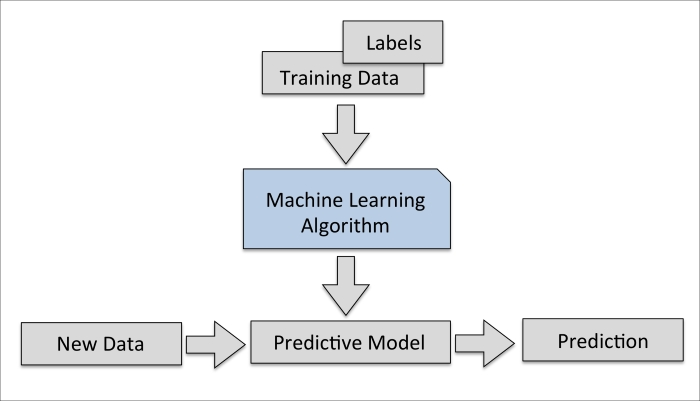#### Classification for predicting class labels

Classification is a subcategory of supervised learning where the goal is to predict the categorical class labels of new instances based on past observations. Those class labels are discrete, unordered values that can be understood as the group memberships of the instances. The previously mentioned example of e-mail-spam detection represents a typical example of a binary classification task, where the machine learning algorithm learns a set of rules in order to distinguish between two possible classes: spam and non-spam e-mail.

However, the set of class labels does not have to be of a binary nature. The predictive model learned by a supervised learning algorithm can assign any class label that was presented in the training dataset to a new, unlabeled instance. A typical example of a multi-class classification task is handwritten character recognition. Here, we could collect a training dataset that consists of multiple handwritten examples of each letter in the alphabet. Now, if a user provides a new handwritten character via an input device, our predictive model will be able to predict the correct letter in the alphabet with certain accuracy. However, our machine learning system would be unable to correctly recognize any of the digits zero to nine, for example, if they were not part of our training dataset.

The following figure illustrates the concept of a binary classification task given 30 training samples: 15 training samples are labeled as negative class (circles) and 15 training samples are labeled as positive class (plus signs). In this scenario, our dataset is two-dimensional, which means that each sample has two values associated with it: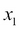and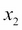. Now, we can use a supervised machine learning algorithm to learn a rule—the decision boundary represented as a black dashed line—that can separate those two classes and classify new data into each of those two categories given itsandvalues: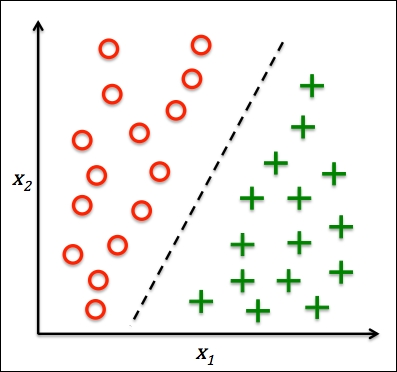#### Regression for predicting continuous outcomes

We learned in the previous section that the task of classification is to assign categorical, unordered labels to instances. A second type of supervised learning is the prediction of continuous outcomes, which is also called regression analysis. In regression analysis, we are given a number of predictor (explanatory) variables and a continuous response variable (outcome), and we try to find a relationship between those variables that allows us to predict an outcome.

For example, let's assume that we are interested in predicting the Math SAT scores of our students. If there is a relationship between the time spent studying for the test and the final scores, we could use it as training data to learn a model that uses the study time to predict the test scores of future students who are planning to take this test.

### Note

The term regression was devised by Francis Galton in his article Regression Towards Mediocrity in Hereditary Stature in 1886. Galton described the biological phenomenon that the variance of height in a population does not increase over time. He observed that the height of parents is not passed on to their children but the children's height is regressing towards the population mean.

The following figure illustrates the concept of linear regression. Given a predictor variable x and a response variable y, we fit a straight line to this data that minimizes the distance—most commonly the average squared distance—between the sample points and the fitted line. We can now use the intercept and slope learned from this data to predict the outcome variable of new data: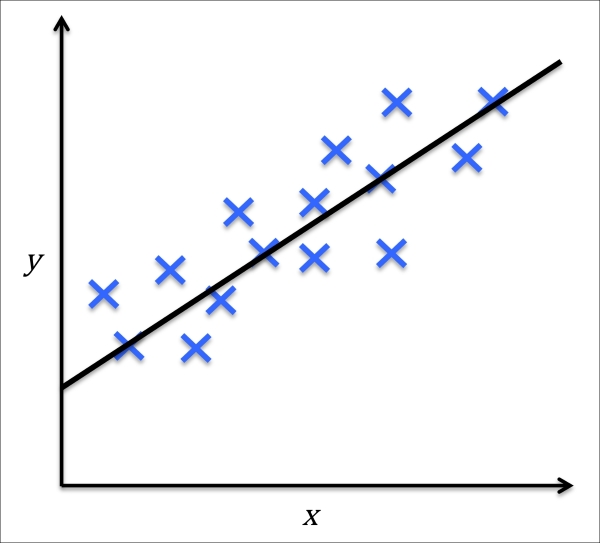### Solving interactive problems with reinforcement learning

Another type of machine learning is reinforcement learning. In reinforcement learning, the goal is to develop a system (agent) that improves its performance based on interactions with the environment. Since the information about the current state of the environment typically also includes a so-called reward signal, we can think of reinforcement learning as a field related to supervised learning. However, in reinforcement learning this feedback is not the correct ground truth label or value, but a measure of how well the action was measured by a reward function. Through the interaction with the environment, an agent can then use reinforcement learning to learn a series of actions that maximizes this reward via an exploratory trial-and-error approach or deliberative planning.

A popular example of reinforcement learning is a chess engine. Here, the agent decides upon a series of moves depending on the state of the board (the environment), and the reward can be defined as win or lose at the end of the game: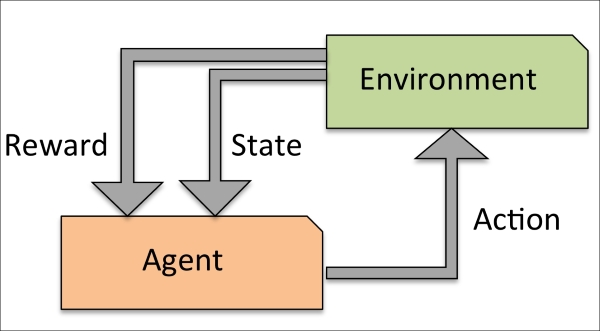### Discovering hidden structures with unsupervised learning

In supervised learning, we know the right answer beforehand when we train our model, and in reinforcement learning, we define a measure of reward for particular actions by the agent. In unsupervised learning, however, we are dealing with unlabeled data or data of unknown structure. Using unsupervised learning techniques, we are able to explore the structure of our data to extract meaningful information without the guidance of a known outcome variable or reward function.

#### Finding subgroups with clustering

Clustering is an exploratory data analysis technique that allows us to organize a pile of information into meaningful subgroups (clusters) without having any prior knowledge of their group memberships. Each cluster that may arise during the analysis defines a group of objects that share a certain degree of similarity but are more dissimilar to objects in other clusters, which is why clustering is also sometimes called "unsupervised classification." Clustering is a great technique for structuring information and deriving meaningful relationships among data, For example, it allows marketers to discover customer groups based on their interests in order to develop distinct marketing programs.

The figure below illustrates how clustering can be applied to organizing unlabeled data into three distinct groups based on the similarity of their featuresand: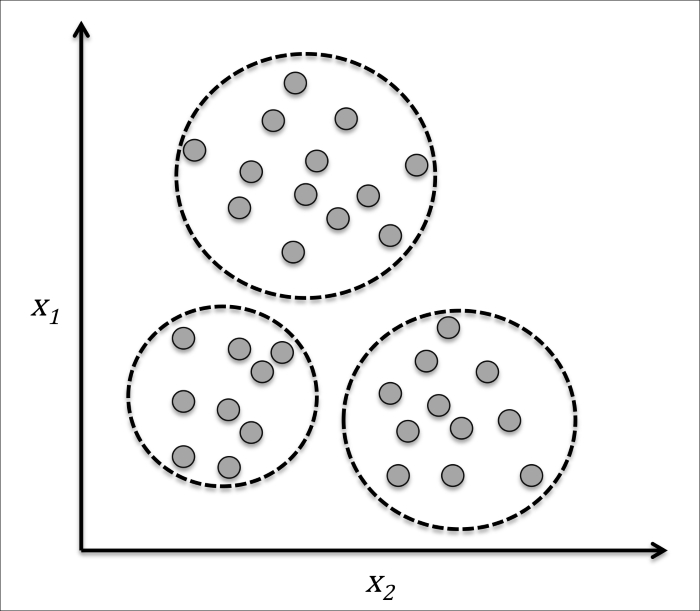#### Dimensionality reduction for data compression

Another subfield of unsupervised learning is dimensionality reduction. Often we are working with data of high dimensionality—each observation comes with a high number of measurements—that can present a challenge for limited storage space and the computational performance of machine learning algorithms. Unsupervised dimensionality reduction is a commonly used approach in feature preprocessing to remove noise from data, which can also degrade the predictive performance of certain algorithms, and compress the data onto a smaller dimensional subspace while retaining most of the relevant information.

Sometimes, dimensionality reduction can also be useful for visualizing data—for example, a high-dimensional feature set can be projected onto one-, two-, or three-dimensional feature spaces in order to visualize it via 3D- or 2D-scatterplots or histograms. The figure below shows an example where non-linear dimensionality reduction was applied to compress a 3D Swiss Roll onto a new 2D feature subspace: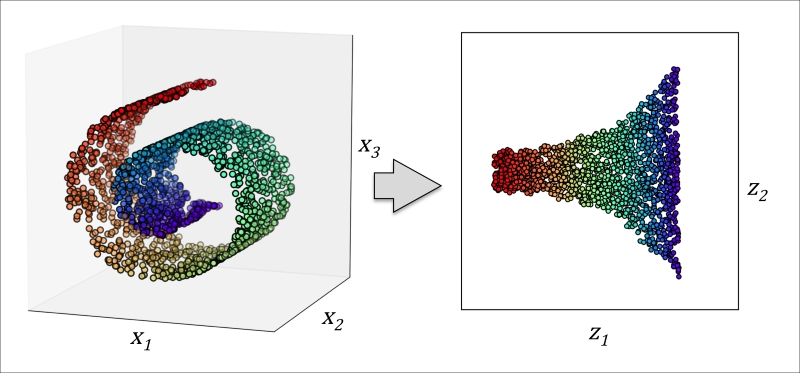## An introduction to the basic terminology and notations

Now that we have discussed the three broad categories of machine learning—supervised, unsupervised, and reinforcement learning—let us have a look at the basic terminology that we will be using in the next chapters. The following table depicts an excerpt of the Iris dataset, which is a classic example in the field of machine learning. The Iris dataset contains the measurements of 150 iris flowers from three different species: Setosa, Versicolor, and Virginica. Here, each flower sample represents one row in our data set, and the flower measurements in centimeters are stored as columns, which we also call the features of the dataset: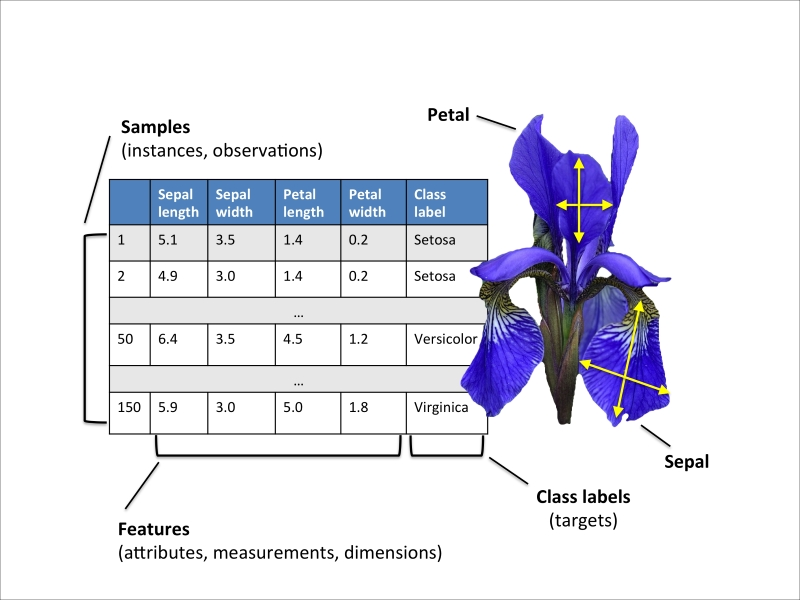To keep the notation and implementation simple yet efficient, we will make use of some of the basics of linear algebra. In the following chapters, we will use a matrix and vector notation to refer to our data. We will follow the common convention to represent each sample as separate row in a feature matrix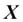, where each feature is stored as a separate column.

The Iris dataset, consisting of 150 samples and 4 features, can then be written as a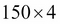matrix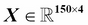: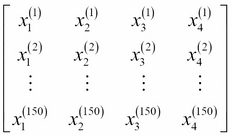### Note

For the rest of this book, we will use the superscript (i) to refer to the ith training sample, and the subscript j to refer to the jth dimension of the training dataset.

We use lower-case, bold-face letters to refer to vectors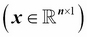and upper-case, bold-face letters to refer to matrices, respectively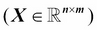. To refer to single elements in a vector or matrix, we write the letters in italics (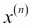or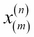, respectively).

For example,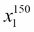refers to the first dimension of flower sample 150, the sepal length. Thus, each row in this feature matrix represents one flower instance and can be written as four-dimensional row vector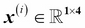,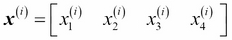.

Each feature dimension is a 150-dimensional column vector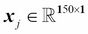, for example: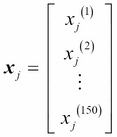.

Similarly, we store the target variables (here: class labels) as a 150-dimensional column vector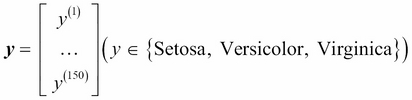.

## A roadmap for building machine learning systems

In the previous sections, we discussed the basic concepts of machine learning and the three different types of learning. In this section, we will discuss other important parts of a machine learning system accompanying the learning algorithm. The diagram below shows a typical workflow diagram for using machine learning in predictive modeling, which we will discuss in the following subsections: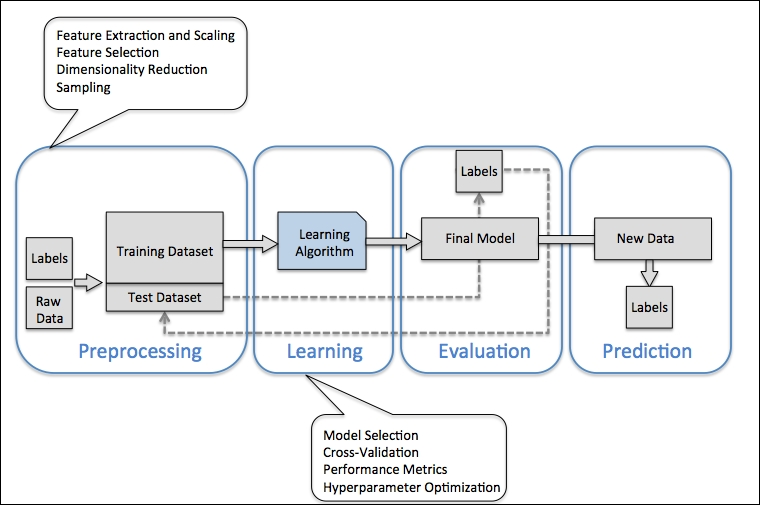### Preprocessing – getting data into shape

Raw data rarely comes in the form and shape that is necessary for the optimal performance of a learning algorithm. Thus, the preprocessing of the data is one of the most crucial steps in any machine learning application. If we take the Iris flower dataset from the previous section as an example, we could think of the raw data as a series of flower images from which we want to extract meaningful features. Useful features could be the color, the hue, the intensity of the flowers, the height, and the flower lengths and widths. Many machine learning algorithms also require that the selected features are on the same scale for optimal performance, which is often achieved by transforming the features in the range [0, 1] or a standard normal distribution with zero mean and unit variance, as we will see in the later chapters.

Some of the selected features may be highly correlated and therefore redundant to a certain degree. In those cases, dimensionality reduction techniques are useful for compressing the features onto a lower dimensional subspace. Reducing the dimensionality of our feature space has the advantage that less storage space is required, and the learning algorithm can run much faster.

To determine whether our machine learning algorithm not only performs well on the training set but also generalizes well to new data, we also want to randomly divide the dataset into a separate training and test set. We use the training set to train and optimize our machine learning model, while we keep the test set until the very end to evaluate the final model.

### Training and selecting a predictive model

As we will see in later chapters, many different machine learning algorithms have been developed to solve different problem tasks. An important point that can be summarized from David Wolpert's famous No Free Lunch Theorems is that we can't get learning "for free" (The Lack of A Priori Distinctions Between Learning Algorithms, D.H. Wolpert 1996; No Free Lunch Theorems for Optimization, D.H. Wolpert and W.G. Macready, 1997). Intuitively, we can relate this concept to the popular saying, "I suppose it is tempting, if the only tool you have is a hammer, to treat everything as if it were a nail" (Abraham Maslow, 1966). For example, each classification algorithm has its inherent biases, and no single classification model enjoys superiority if we don't make any assumptions about the task. In practice, it is therefore essential to compare at least a handful of different algorithms in order to train and select the best performing model. But before we can compare different models, we first have to decide upon a metric to measure performance. One commonly used metric is classification accuracy, which is defined as the proportion of correctly classified instances.

One legitimate question to ask is: how do we know which model performs well on the final test dataset and real-world data if we don't use this test set for the model selection but keep it for the final model evaluation? In order to address the issue embedded in this question, different cross-validation techniques can be used where the training dataset is further divided into training and validation subsets in order to estimate the generalization performance of the model. Finally, we also cannot expect that the default parameters of the different learning algorithms provided by software libraries are optimal for our specific problem task. Therefore, we will make frequent use of hyperparameter optimization techniques that help us to fine-tune the performance of our model in later chapters. Intuitively, we can think of those hyperparameters as parameters that are not learned from the data but represent the knobs of a model that we can turn to improve its performance, which will become much clearer in later chapters when we see actual examples.

### Evaluating models and predicting unseen data instances

After we have selected a model that has been fitted on the training dataset, we can use the test dataset to estimate how well it performs on this unseen data to estimate the generalization error. If we are satisfied with its performance, we can now use this model to predict new, future data. It is important to note that the parameters for the previously mentioned procedures—such as feature scaling and dimensionality reduction—are solely obtained from the training dataset, and the same parameters are later re-applied to transform the test dataset, as well as any new data samples—the performance measured on the test data may be overoptimistic otherwise.

## Using Python for machine learning

Python is one of the most popular programming languages for data science and therefore enjoys a large number of useful add-on libraries developed by its great community.

Although the performance of interpreted languages, such as Python, for computation-intensive tasks is inferior to lower-level programming languages, extension libraries such as NumPy and SciPy have been developed that build upon lower layer Fortran and C implementations for fast and vectorized operations on multidimensional arrays.

For machine learning programming tasks, we will mostly refer to the scikit-learn library, which is one of the most popular and accessible open source machine learning libraries as of today.

### Installing Python packages

Python is available for all three major operating systems—Microsoft Windows, Mac OS X, and Linux—and the installer, as well as the documentation, can be downloaded from the official Python website: https://www.python.org.

This book is written for Python version >= 3.4.3, and it is recommended you use the most recent version of Python 3 that is currently available, although most of the code examples may also be compatible with Python >= 2.7.10. If you decide to use Python 2.7 to execute the code examples, please make sure that you know about the major differences between the two Python versions. A good summary about the differences between Python 3.4 and 2.7 can be found at https://wiki.python.org/moin/Python2orPython3.

The additional packages that we will be using throughout this book can be installed via the pip installer program, which has been part of the Python standard library since Python 3.3. More information about pip can be found at https://docs.python.org/3/installing/index.html.

After we have successfully installed Python, we can execute pip from the command line terminal to install additional Python packages:

```pip install SomePackage
```

Already installed packages can be updated via the `--upgrade` flag:

```pip install SomePackage --upgrade
```

A highly recommended alternative Python distribution for scientific computing is Anaconda by Continuum Analytics. Anaconda is a free—including commercial use—enterprise-ready Python distribution that bundles all the essential Python packages for data science, math, and engineering in one user-friendly cross-platform distribution. The Anaconda installer can be downloaded at http://continuum.io/downloads#py34, and an Anaconda quick start-guide is available at https://store.continuum.io/static/img/Anaconda-Quickstart.pdf.

After successfully installing Anaconda, we can install new Python packages using the following command:

```conda install SomePackage
```

Existing packages can be updated using the following command:

```conda update SomePackage
```

Throughout this book, we will mainly use NumPy's multi-dimensional arrays to store and manipulate data. Occasionally, we will make use of pandas, which is a library built on top of NumPy that provides additional higher level data manipulation tools that make working with tabular data even more convenient. To augment our learning experience and visualize quantitative data, which is often extremely useful to intuitively make sense of it, we will use the very customizable matplotlib library.

The version numbers of the major Python packages that were used for writing this book are listed below. Please make sure that the version numbers of your installed packages are equal to, or greater than, those version numbers to ensure the code examples run correctly:

• NumPy 1.9.1

• SciPy 0.14.0

• scikit-learn 0.15.2

• matplotlib 1.4.0

• pandas 0.15.2

## Summary

In this chapter, we explored machine learning on a very high level and familiarized ourselves with the big picture and major concepts that we are going to explore in the next chapters in more detail.

We learned that supervised learning is composed of two important subfields: classification and regression. While classification models allow us to categorize objects into known classes, we can use regression analysis to predict the continuous outcomes of target variables. Unsupervised learning not only offers useful techniques for discovering structures in unlabeled data, but it can also be useful for data compression in feature preprocessing steps.

We briefly went over the typical roadmap for applying machine learning to problem tasks, which we will use as a foundation for deeper discussions and hands-on examples in the following chapters. Eventually, we set up our Python environment and installed and updated the required packages to get ready to see machine-learning in action.

In the following chapter, we will implement one of the earliest machine learning algorithms for classification that will prepare us for Chapter 3, A Tour of Machine Learning Classifiers Using Scikit-learn, where we cover more advanced machine learning algorithms using the scikit-learn open source machine learning library. Since machine learning algorithms learn from data, it is critical that we feed them useful information, and in Chapter 4, Building Good Training Sets—Data Preprocessing we will take a look at important data preprocessing techniques. In Chapter 5, Compressing Data via Dimensionality Reduction, we will learn about dimensionality reduction techniques that can help us to compress our dataset onto a lower-dimensional feature subspace, which can be beneficial for computational efficiency. An important aspect of building machine learning models is to evaluate their performance and to estimate how well they can make predictions on new, unseen data. In Chapter 6, Learning Best Practices for Model Evaluation and Hyperparameter Tuning we will learn all about the best practices for model tuning and evaluation. In certain scenarios, we still may not be satisfied with the performance of our predictive model although we may have spent hours or days extensively tuning and testing. In Chapter 7, Combining Different Models for Ensemble Learning we will learn how to combine different machine learning models to build even more powerful predictive systems.

After we covered all of the important concepts of a typical machine learning pipeline, we will implement a model for predicting emotions in text in Chapter 8, Applying Machine Learning to Sentiment Analysis, and in Chapter 9, Embedding a Machine Learning Model into a Web Application, we will embed it into a Web application to share it with the world. In Chapter 10, Predicting Continuous Target Variables with Regression Analysis we will then use machine learning algorithms for regression analysis that allow us to predict continuous output variables, and in Chapter 11, Working with Unlabelled Data – Clustering Analysis we will apply clustering algorithms that will allow us to find hidden structures in data. The last two chapters in this book will cover artificial neural networks that will allow us to tackle complex problems, such as image and speech recognition, which is currently one of the hottest topics in machine-learning research.